4.9.2 Traversal by Iteration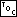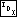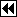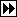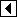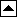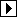The recursive approach of the previous section is one valid way to traverse a binary search tree in sorted order. This method has the advantages of being simple and “obviously correct”. But it does have problems with efficiency, because each call to traverse_recursive() receives its own duplicate copies of arguments action and param, and with convenience, because writing a new callback function for each traversal is unpleasant. It has other problems, too, as already discussed, but these are the ones to be addressed immediately.

The simplest way to eliminate recursion is by a literal conversion of the recursion to iteration. This is the topic of this section. Later, we will consider a slightly different, and in some ways superior, iterative solution.

Converting recursion into iteration is an interesting problem. There are two main ways to do it:

tail recursion elimination
If a recursive call is the last action taken in a function, then it is equivalent to a goto back to the beginning of the function, possibly after modifying argument values. (If the function has a return value then the recursive call must be a return statement returning the value received from the nested call.) This form of recursion is called tail recursion (see tail recursion).
save-and-restore recursion elimination
In effect, a recursive function call saves a copy of argument values and local variables, modifies the arguments, then executes a goto to the beginning of the function. Accordingly, the return from the nested call is equivalent to restoring the saved arguments and local variables, then executing a goto back to the point where the call was made.

We can make use of both of these rules in converting traverse_recursive() to iterative form. First, does traverse_recursive() ever call itself as its last action? The answer is yes, so we can convert that to an assignment plus a goto statement:

```51. <Iterative traversal of BST, take 1 51> =
static void traverse_iterative (struct bst_node *node, bst_item_func *action, void *param) {
start:
if (node != NULL)     {
traverse_iterative (node->bst_link, action, param);
action (node->bst_data, param);
goto start;
}
}
```

Sensible programmers are not fond of goto. Fortunately, it is easy to eliminate by rephrasing in terms of a while loop:

```52. <Iterative traversal of BST, take 2 52> =
static void traverse_iterative (struct bst_node *node, bst_item_func *action, void *param) {
while (node != NULL)     {
traverse_iterative (node->bst_link, action, param);
action (node->bst_data, param);
}
}
```

This still leaves another recursive call, one that is not tail recursive. This one must be eliminated by saving and restoring values. A stack is ideal for this purpose. For now, we use a stack of fixed size BST_MAX_HEIGHT and deal with stack overflow by aborting. Later, we'll handle overflow more gracefully. Here's the code:

```53. <Iterative traversal of BST, take 3 53> =
static void traverse_iterative (struct bst_node *node, bst_item_func *action, void *param) {
struct bst_node *stack[BST_MAX_HEIGHT];
size_t height = 0;

start:
while (node != NULL)     {
if (height >= BST_MAX_HEIGHT)         {
fprintf (stderr, "tree too deep\n");
exit (EXIT_FAILURE);
}
stack[height++] = node;
goto start;

resume:
action (node->bst_data, param);
}

if (height > 0)     {
node = stack[–height];
goto resume;
}
}
```

This code, an ugly mash of statements, is a prime example of why goto statements are discouraged, but its relationship with the earlier code is clear. To make it acceptable for real use, we must rephrase it. First, we can eliminate label resume by recognizing that it can only be reached from the corresponding goto statement, then moving its code appropriately:

```54. <Iterative traversal of BST, take 4 54> =
static void traverse_iterative (struct bst_node *node, bst_item_func *action, void *param) {
struct bst_node *stack[BST_MAX_HEIGHT];
size_t height = 0;

start:
while (node != NULL)     {
if (height >= BST_MAX_HEIGHT)         {
fprintf (stderr, "tree too deep\n");
exit (EXIT_FAILURE);
}
stack[height++] = node;
goto start;
}

if (height > 0)     {
node = stack[–height];
action (node->bst_data, param);
goto start;
}
}
```

The first remaining goto statement can be eliminated without any other change, because it is redundant; the second, by enclosing the whole function body in an “infinite loop”:

```55. <Iterative traversal of BST, take 5 55> =
static void traverse_iterative (struct bst_node *node, bst_item_func *action, void *param) {
struct bst_node *stack[BST_MAX_HEIGHT];
size_t height = 0;

for (;;)     {
while (node != NULL)         {
if (height >= BST_MAX_HEIGHT)             {
fprintf (stderr, "tree too deep\n");
exit (EXIT_FAILURE);
}
stack[height++] = node;
}

if (height == 0)
break;

node = stack[–height];
action (node->bst_data, param);
}
}
```

This initial iterative version takes care of the efficiency problem.

Exercises:

1. Function traverse_iterative() relies on stack[], a stack of nodes yet to be visited, which as allocated can hold up to BST_MAX_HEIGHT nodes. Consider the following questions concerning stack[]:

1. What is the maximum height this stack will attain in traversing a binary search tree containing n nodes, if the binary tree has minimum possible height?
2. What is the maximum height this stack can attain in traversing any binary tree of n nodes? The minimum height?
3. Under what circumstances is it acceptable to use a fixed-size stack as in the example code?
4. Rewrite traverse_iterative() to dynamically expand stack[] in case of overflow.
5. Does traverse_recursive() also have potential for running out of “stack” or “memory”? If so, more or less than traverse_iterative() as modified by the previous part?